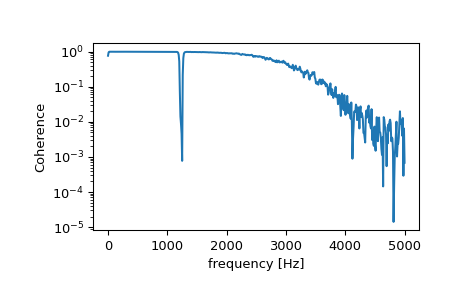# scipy.signal.coherence¶

scipy.signal.coherence(x, y, fs=1.0, window='hann', nperseg=None, noverlap=None, nfft=None, detrend='constant', axis=- 1)[source]

Estimate the magnitude squared coherence estimate, Cxy, of discrete-time signals X and Y using Welch’s method.

`Cxy = abs(Pxy)**2/(Pxx*Pyy)`, where Pxx and Pyy are power spectral density estimates of X and Y, and Pxy is the cross spectral density estimate of X and Y.

Parameters
xarray_like

Time series of measurement values

yarray_like

Time series of measurement values

fsfloat, optional

Sampling frequency of the x and y time series. Defaults to 1.0.

windowstr or tuple or array_like, optional

Desired window to use. If window is a string or tuple, it is passed to `get_window` to generate the window values, which are DFT-even by default. See `get_window` for a list of windows and required parameters. If window is array_like it will be used directly as the window and its length must be nperseg. Defaults to a Hann window.

npersegint, optional

Length of each segment. Defaults to None, but if window is str or tuple, is set to 256, and if window is array_like, is set to the length of the window.

noverlap: int, optional

Number of points to overlap between segments. If None, `noverlap = nperseg // 2`. Defaults to None.

nfftint, optional

Length of the FFT used, if a zero padded FFT is desired. If None, the FFT length is nperseg. Defaults to None.

detrendstr or function or False, optional

Specifies how to detrend each segment. If `detrend` is a string, it is passed as the type argument to the `detrend` function. If it is a function, it takes a segment and returns a detrended segment. If `detrend` is False, no detrending is done. Defaults to ‘constant’.

axisint, optional

Axis along which the coherence is computed for both inputs; the default is over the last axis (i.e. `axis=-1`).

Returns
fndarray

Array of sample frequencies.

Cxyndarray

Magnitude squared coherence of x and y.

`periodogram`

Simple, optionally modified periodogram

`lombscargle`

Lomb-Scargle periodogram for unevenly sampled data

`welch`

Power spectral density by Welch’s method.

`csd`

Cross spectral density by Welch’s method.

Notes

An appropriate amount of overlap will depend on the choice of window and on your requirements. For the default Hann window an overlap of 50% is a reasonable trade off between accurately estimating the signal power, while not over counting any of the data. Narrower windows may require a larger overlap.

New in version 0.16.0.

References

1

P. Welch, “The use of the fast Fourier transform for the estimation of power spectra: A method based on time averaging over short, modified periodograms”, IEEE Trans. Audio Electroacoust. vol. 15, pp. 70-73, 1967.

2

Stoica, Petre, and Randolph Moses, “Spectral Analysis of Signals” Prentice Hall, 2005

Examples

```>>> from scipy import signal
>>> import matplotlib.pyplot as plt
>>> rng = np.random.default_rng()
```

Generate two test signals with some common features.

```>>> fs = 10e3
>>> N = 1e5
>>> amp = 20
>>> freq = 1234.0
>>> noise_power = 0.001 * fs / 2
>>> time = np.arange(N) / fs
>>> b, a = signal.butter(2, 0.25, 'low')
>>> x = rng.normal(scale=np.sqrt(noise_power), size=time.shape)
>>> y = signal.lfilter(b, a, x)
>>> x += amp*np.sin(2*np.pi*freq*time)
>>> y += rng.normal(scale=0.1*np.sqrt(noise_power), size=time.shape)
```

Compute and plot the coherence.

```>>> f, Cxy = signal.coherence(x, y, fs, nperseg=1024)
>>> plt.semilogy(f, Cxy)
>>> plt.xlabel('frequency [Hz]')
>>> plt.ylabel('Coherence')
>>> plt.show()
```